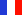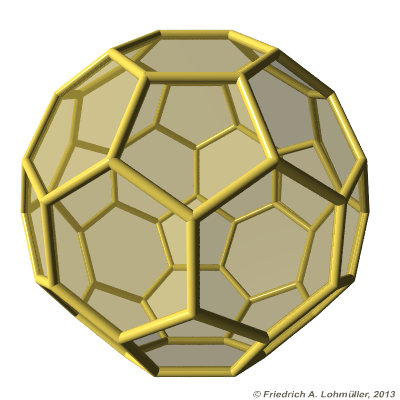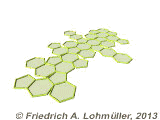###### Descriptions and Examples for the POV-Ray Raytracerby Friedrich A. Lohmüller Elementary Geometry for Raytracing
ItalianoFrançaisDeutschHome
- POV-Ray Tutorial

- Geometrical Basics
for Raytracing

Right-angled Triangle
Pythagorean Theorem
Trigonometry Basics
Law of cosines
Equilateral Triangle
Regular Polygon
Polyhedron
Tetrahedron
Octahedron
Cube & Cuboid
Dodecahedron
Icosahedron
Cuboctahedron
Truncated Octahedron
Rhombicuboctahedron
Truncated Icosahedron
Circles
Tangent circles
Internal Tangents
External Tangents

- Geometric 3D Animations## Truncated Icosahedron Buckminsterfullerene buckyball, C60.Some useful geometrical facts.Truncated Icosahedron
In the following we write for the square root of a number
the expression "sqrt(
ZAHL)"
conforming to the syntax used in POV-Ray.

 Dimensions Length of an edge: a. Radius of circumsphere (vertices): Rc = a / 4 * sqrt( 58 + 18*sqrt(5)); Radius of edgesphere (tangent to edges): Re = 3/4 * a * ( 1 + sqrt(5) ); Angle between hexagon and hexagon: ~138,2° HH_Angle = degrees(acos(-1/3*sqrt(5))); Angle between hexagon and pentagon: ~142,6° HP_Angle = degrees(acos(-sqrt((5+2*sqrt(5))/15)));Truncated Icosahedron netTruncated IcosahedronFolding of a Truncated Icosahedron
top

 © Friedrich A. Lohmüller, 2013 www.f-lohmueller.de